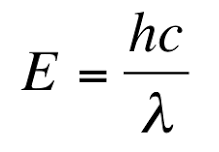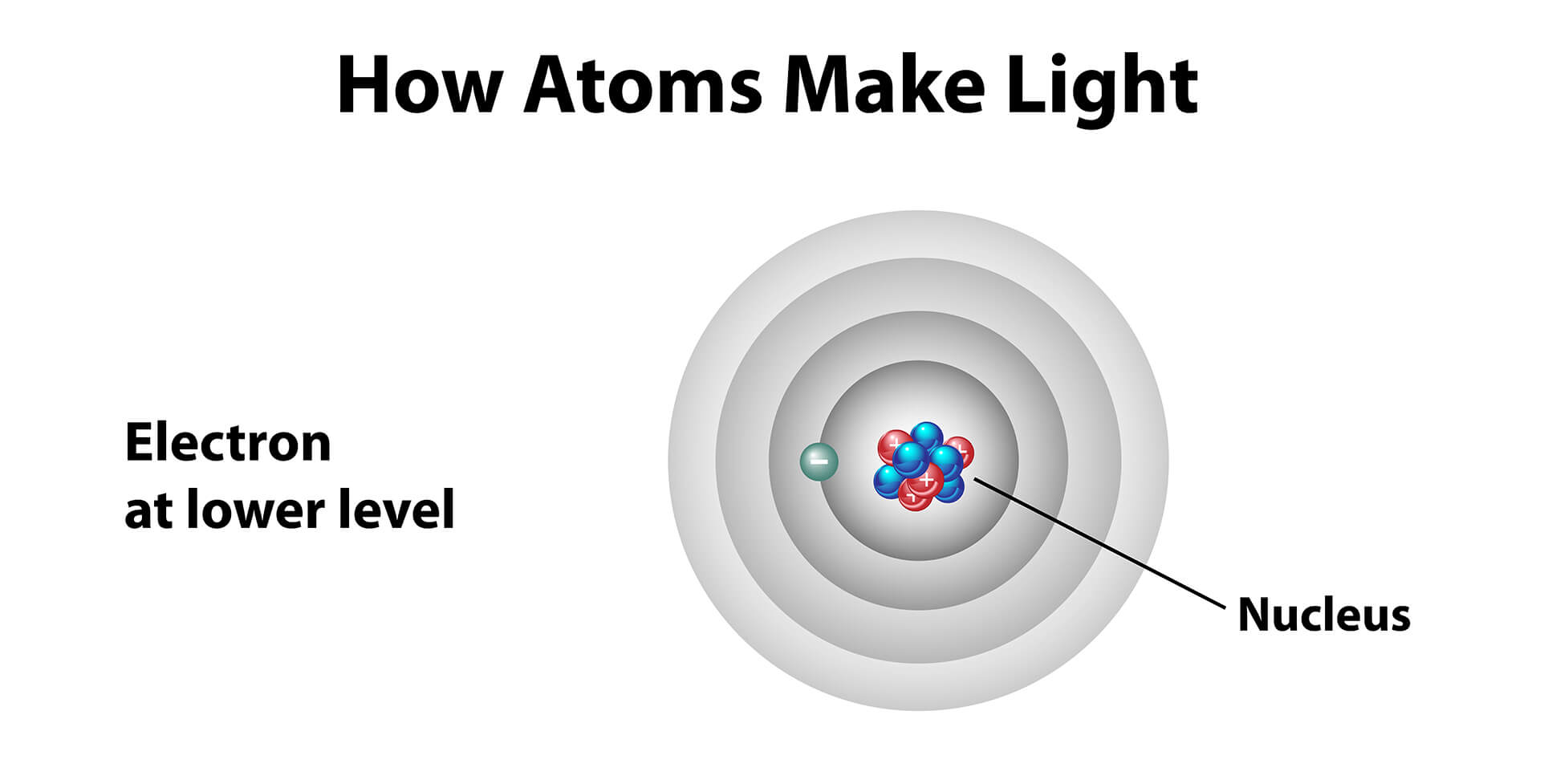## Lesson 14 – How do Atoms Emit Light?

Posted on: Tuesday, July 12th, 2022  In: Learning Optics with Austin

Light sources such as the sun give off light, but why? The equation E = hf relates the wavelength of light to energy where E is energy, h is Planck’s constant, and f is the frequency. Since we know from “How to Describe Light” that f = c / λ, we can also rewrite the equation into E = hc / λ. Thus, as λ (aka the wavelength) increases, energy decreases. From this relationship, we observe that the wavelength of light is related to its energy levels.Rewriting the Planck Relation

To understand how different energy levels relate to light sources, we must look at matter at the atomic level. In the planetary model of the atom, electrons orbit a nucleus in rings. Each ring can be thought of as an energy level where electrons closest to the nucleus are at the lowest energy level and electrons farthest away from the nucleus are at the highest energy level. Electrons further away from the nucleus are also more unstable.Planetary Model of the Atom

Suppose that an atom has 2 rings and that there is a single electron on the outer ring. If the electron were to drop from the outer ring with an energy level of E2 to the inner ring with an energy level of E1, then the change of energy can be written as Δ E = E2 – E1. Since energy is neither created or destroyed (the law of conservation of energy), the loss of energy must go somewhere. In fact, the energy is released as a photon which is what gives off light.Absorption and Emission

If the photon is represented by ΔE, then substituting it into E = hf and rearranging the terms results in f = (E2 – E1) / h. The significance of this equation is that it relates the color of the light being emitted to the energy of the photon! As ΔE gets larger (the change in energy levels), the frequency of light also increases which trends towards the UV end of the visible light spectrum. As ΔE gets smaller, the frequency of light decreases which trends towards the infrared end of the visible light spectrum.

Next: TBD